# 2845. 符号方程求解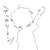##### Python Code
s=input()
p=0
while 1:
if s[p]!='+' and s[p]!='-' and s[p]!='=' and not s[p].isdigit():
a=s[p]
break
p+=1
s=s.replace(a,'x')
p=1
while p!=len(s):
if s[p-1].isdigit() and s[p]=='x':
s=s[:p]+'*'+s[p:]
p+=1
p=s.find('=')
s=s[:p]+'-('+s[p+1:]+')'
x=0
t=eval(s)#真·核弹炸水题。emmm~
x=1
print(a+('=%.3f'%(t/(t-eval(s))) if t!=0 else '=0.000'))
#0为解巨坑！虽然可以四舍五入但小负数四舍五入后会变成-0.000！（那啥，精度问题……）
#看了大佬提示还过了很久才发现是真的菜……


ps:蟒蛇NB！
pps:C&C++什么的忘了吧！以后是玩蛇的时代！人生苦短，__。
ppps:西神这学期又变帅(qiang)了，hello新学期！
pppps:以上彩蛋只有聪明人能看见。（逃~）
ppppps:彩蛋内容本条除外，其余均大雾。（题外话：这好像是我第一次评论区贴完整代码？纪念一下。以及：空了这么多行你们是不是都发现彩蛋了。233~）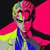#include<bits/stdc++.h>
using namespace std;
int sum1=0,sum2=0;
{
sum1=0,sum2=0;
//sum1存未知数前面的系数,sum2存已知数的总和。
int i=0,sign=1,ceof=0;
while(s[i])
{
sign=1,ceof=0;
if(s[i]=='-'){
sign=-1;i++;
}
else if(s[i]=='+'){
sign=1;i++;
}
if(s[i]>='0'&&s[i]<='9'){
while(s[i]>='0'&&s[i]<='9'){
ceof=ceof*10+s[i]-'0';
i++;
}
if(s[i]>='a'&&s[i]<='z'){
sum1+=(sign*ceof);
i++;
}
else{
sum2+=(sign*ceof);
}
}
else if(s[i]>='a'&&s[i]<='z'){
ceof=1;
sum1+=(sign*ceof);
i++;
}
}
}
int main()
{
string s,s1,s2;
cin>>s;char ch;
for(int i=0;i<s.size();i++){
if(s[i]>='a'&&s[i]<='z'){
ch=s[i];break;
}
}
s1=s.substr(0,s.find("="));
s2=s.substr(s.find("=")+1,s.size()-s.find("=")-1);
int ceof_unknown_left=sum1;
int ceof_known_left=sum2;
int ceof_unknown_right=sum1;
int ceof_known_right=sum2;
int aa=ceof_unknown_left-ceof_unknown_right;
int bb=ceof_known_right-ceof_known_left;
double x=bb*1.0/aa*1.0;
printf("%c=%.3lf",ch,x);
}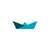#include <iostream>
#include <vector>
#include <string>
#include <algorithm>
using namespace std;
int main(){
char varied = '%';
vector<int> position;
int leftnum = 0, rightnum = 0;
string input, temp;
getline(cin, input);
int equalPos = input.find('=');
int pos = input.find('+', 0);
while (input.find('+', pos) != string::npos){
position.push_back(input.find('+', pos));
pos = input.find('+', pos + 1);
}
pos = input.find('-', 0);
while (input.find('-', pos) != string::npos){
position.push_back(input.find('-', pos));
pos = input.find('-', pos + 1);
}
position.push_back(equalPos);
position.push_back(0);
position.push_back(input.length());
sort(position.begin(), position.end());
for (int i = 0; i <= position.size()-1; i++){
string temp;
temp = input.substr(position[i], position[i + 1] - position[i]);
if(position[i]<equalPos){
if (temp.length() == 0)
;
else if (temp[temp.length() - 1] >= 'a' && temp[temp.length() - 1] <= 'z'){
varied = temp[temp.length() - 1];
if (temp.length() == 1 || (temp.length()==2 && temp=='+'))
leftnum += 1;
else if(temp.length()==2&&temp=='-')
leftnum -= 1;
else
leftnum += atoi((temp.substr(0, temp.length() - 1)).c_str());
}
else{
rightnum -= atoi(temp.c_str());
}
}
else if (position[i] == equalPos){
position[i]++;
i--;
continue;
}
else{
if (temp.length() == 0)
;
else if (temp[temp.length() - 1] >= 'a' && temp[temp.length() - 1] <= 'z'){
varied = temp[temp.length() - 1];
if (temp.length() == 1 || (temp.length()==2 && temp=='+'))
leftnum -= 1;
else if(temp.length()==2&&temp=='-')
leftnum += 1;
else
leftnum -= atoi((temp.substr(0, temp.length() - 1)).c_str());
}
else{
rightnum += atoi(temp.c_str());
}
}
}
printf("%c=%.3f", varied, (double)rightnum / (double)leftnum);
return 0;
}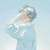EOJ 2821
EOJ 2891
EOJ 2845（本题）

### include

using namespace std;
string strs;
int LA,LN,RA,RN;
char Alpha;
int main()
{
cin>>strs;
int Epos=strs.find(“=”);
int pos=0;
while(pos<Epos)
{
int symbol=1;
if(strs[pos]==’+’) pos++;
else if(strs[pos]==’-‘)
{
++pos;
symbol=-1;
}
int num=-1;
while(pos<Epos && isdigit(strs[pos]))
{
if(num==-1) num=strs[pos]-‘0’;
else num=num10+strs[pos]-‘0’;
pos++;
}
if(num==-1) num=1;
if(isalpha(strs[pos]))
{
Alpha=strs[pos];
LA+=num
symbol;
++pos;
}
else LN+=numsymbol;
}
pos++;
Epos=strs.length();
while(pos<Epos)
{
int symbol=1;
if(strs[pos]==’+’) pos++;
else if(strs[pos]==’-‘)
{
++pos;
symbol=-1;
}
int num=-1;
while(pos<Epos && isdigit(strs[pos]))
{
if(num==-1) num=strs[pos]-‘0’;
else num=num
10+strs[pos]-‘0’;
pos++;
}
if(num==-1) num=1;
if(isalpha(strs[pos]))
{
Alpha=strs[pos];
RA+=numsymbol;
++pos;
}
else RN+=num
symbol;
}
printf(“%c=%.3f\n”,Alpha,(RN-LN)*1.0/(LA-RA));
return 0;
}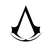c++ string牛批！

### include

using namespace std;
int main()
{
char s;
scanf(“%s”,s);
int l=strlen(s);
int where=0;//to judge if it’s at the left or right
int sqr=1;//to judge whether plus or submit
double sumnum=0;//the number
double suma=0;//the number of a
char c;//the ‘X’
int lin=0;//number to calculate

for(int i=0;i<l;i++)
{
if(s[i]>='a'&&s[i]<='z')
{c=s[i];

if(s[i-1]<'0'||s[i-1]>'9') lin=1;
if(lin!=0)
suma+=lin*sqr;
lin=0;
}

if((s[i]<'0'||s[i]>'9')&&lin!=0){
sumnum=sumnum+lin*sqr;

lin=0;
sqr=1;
//cout<<sumnum<<endl;
}
if(s[i]=='=') where=1,sumnum*=-1,suma*=-1,sqr=1;//cout<<sumnum<<ends<<suma<<endl;
if(s[i]=='+') sqr=1;
if(s[i]=='-') sqr=-1;

if(s[i]>='0'&&s[i]<='9'){
lin=lin*10+s[i]-'0';
//cout<<sqr<<ends<<lin<<endl;
}

}
//for(int i=0;i<l;i++)
// cout<<s[i];

if(lin!=0) sumnum+=lin*sqr;
if(sumnum*-1==0) sumnum=0;
//cout<<sumnum<<ends<<suma<<endl;
suma*=-1;
printf("%c=%.3f\n",c,sumnum/suma);
//cout<<sumnum<<endl;
// cout<<suma<<endl;


}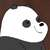### include

using namespace std;
void f(vector &v1,vector &v2,string x,char &c) {
int i=0;
while(i=‘0’&&x[j]<=‘9’) {
sum=sum10+x[j]-‘0’;
j++;
}
if(x[j]>=’a’&&x[j]<=’z’) {
c=x[j];
if(sum==0)
v1.push_back(flag);
else
v1.push_back(flag
sum);
j++;
} else {
v2.push_back(sum*flag);
}
i=j;
}
}
int main() {
string s;
cin>>s;
vectorv1,v2,v3,v4;
int index=s.find(“=”);
string x=s.substr(0,index);
string y=s.substr(index+1,s.size());
char c;
f(v1,v2,x,c);
f(v3,v4,y,c);
int sum1=0;
int sum2=0;
for(int i = 0; i < v1.size(); i++)
sum1+=v1[i];
for(int i = 0; i < v3.size(); i++)
sum1-=v3[i];
for(int i = 0; i < v2.size(); i++)
sum2-=v2[i];
for(int i = 0; i < v4.size(); i++)
sum2+=v4[i];
printf(“%c=%.3f\n”,c,(float)sum2/sum1);
return 0;
}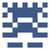#include <iostream>
#include <string>
#include <iomanip>
using namespace std;

double coefA = 0, coefB = 0;
char x;

void compute(string str,bool flag)
{
bool tag = 0;//默认为常数
for (int i = 0;i < str.size();i++) { if (str[i] >= 'a'&&str[i] <= 'z') { x = str[i];tag = 1; } }
if (flag == 0)
{
if (tag == 1 && str.size() == 1) { coefA += 1; }
else if (tag == 1 && str.size() == 2 && str == '+') { coefA += 1; }
else if (tag == 1 && str.size() == 2 && str == '-') { coefA -= 1; }
else if (tag == 1) { coefA += stoi(str); }
if (tag == 0) { coefB += stoi(str); }
}
else
{
if (tag == 1 && str.size() == 1) { coefA -= 1; }
else if (tag == 1 && str.size() == 2 && str == '+') { coefA -= 1; }
else if (tag == 1 && str.size() == 2 && str == '-') { coefA += 1; }
else if (tag == 1) { coefA -= stoi(str); }
if (tag == 0) { coefB -= stoi(str); }
}
}

int main()
{
string s, str="";
cin >> s;
bool flag = 0;//默认为等号前端
for (int i = 0;i < s.size();i++)
{
str += s[i];
if ((s[i + 1] == '+' || s[i + 1] == '-') && flag == 0) { compute(str,flag);str = ""; }
else if ((s[i + 1] == '+' || s[i + 1] == '-') && flag == 1) { compute(str, flag);str = ""; }
if (s[i + 1] == '=') { compute(str, flag);flag = 1;str = "";i++; }
}
if (str != "") { compute(str, flag); }
cout << x << "=";
cout << fixed << setprecision(3)<< coefB / -coefA << endl;
}#include <iostream>
#include <string>
#include <iomanip>
using namespace std;

double coefA = 0, coefB = 0;
char x;

void compute(string str,bool flag)
{
bool tag = 0;//默认为常数
for (int i = 0;i < str.size();i++) { if (str[i] >= 'a'&&str[i] <= 'z') { x = str[i];tag = 1; } }
if (flag == 0)
{
if (tag == 1 && str.size() == 1) { coefA += 1; }
else if (tag == 1 && str.size() == 2 && str == '+') { coefA += 1; }
else if (tag == 1 && str.size() == 2 && str == '-') { coefA -= 1; }
else if (tag == 1) { coefA += stoi(str); }
if (tag == 0) { coefB += stoi(str); }
}
else
{
if (tag == 1 && str.size() == 1) { coefA -= 1; }
else if (tag == 1 && str.size() == 2 && str == '+') { coefA -= 1; }
else if (tag == 1 && str.size() == 2 && str == '-') { coefA += 1; }
else if (tag == 1) { coefA -= stoi(str); }
if (tag == 0) { coefB -= stoi(str); }
}
}

int main()
{
string s, str="";
cin >> s;
bool flag = 0;//默认为等号前端
for (int i = 0;i < s.size();i++)
{
str += s[i];
if ((s[i + 1] == '+' || s[i + 1] == '-') && flag == 0) { compute(str,flag);str = ""; }
else if ((s[i + 1] == '+' || s[i + 1] == '-') && flag == 1) { compute(str, flag);str = ""; }
if (s[i + 1] == '=') { compute(str, flag);flag = 1;str = "";i++; }
}
if (str != "") { compute(str, flag); }
cout << x << "=";
cout << fixed << setprecision(3)<< coefB / -coefA << endl;
}